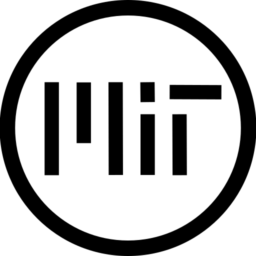# Outline that disrespects boundaries

A rounded edge 2D outline that makes up space for itself in the texture instead of being cut off by the image’s boundaries. No pulsing effects or animation, for when you want a simple outline that Just Works! You can choose a color, line thickness, and whether or not to add margins.

Works better with higher-res images, not meant for pixel art.

Warning: you need to have at least 1 pixel of separation between the image’s content and its edges for the margins to work properly. If you import your images with mipmapping on, you might need to add a bit more space.

Simple modification of this GDQuest shader with portions of this other shader by Juulpower.

``````shader_type canvas_item;

uniform vec4 line_color : hint_color = vec4(1);
uniform float line_thickness : hint_range(0, 20) = 0.0;

void vertex() {
VERTEX += (UV * 2.0 - 1.0) * line_thickness;
}
}

void fragment() {
vec2 uv = UV;

vec2 texture_pixel_size = vec2(1.0) / (vec2(1.0) / TEXTURE_PIXEL_SIZE + vec2(line_thickness * 2.0));

uv = (uv - texture_pixel_size * line_thickness) * TEXTURE_PIXEL_SIZE / texture_pixel_size;

if (uv != clamp(uv, vec2(0.0), vec2(1.0))) {
COLOR.a = 0.0;
} else {
COLOR = texture(TEXTURE, uv);
}
} else {
COLOR = texture(TEXTURE, uv);
}

vec2 size = TEXTURE_PIXEL_SIZE * line_thickness;

if (line_thickness < 0.1) {
vec4 color = texture(TEXTURE, uv);
COLOR = color;
} else {
float outline = texture(TEXTURE, uv + vec2(-size.x, 0)).a;
outline += texture(TEXTURE, uv + vec2(0, size.y)).a;
outline += texture(TEXTURE, uv + vec2(size.x, 0)).a;
outline += texture(TEXTURE, uv + vec2(0, -size.y)).a;
outline += texture(TEXTURE, uv + vec2(-size.x * 0.866, size.y * 0.5)).a;
outline += texture(TEXTURE, uv + vec2(-size.x * 0.5, size.y * 0.866)).a;
outline += texture(TEXTURE, uv + vec2(size.x * 0.866, size.y * 0.5)).a;
outline += texture(TEXTURE, uv + vec2(size.x * 0.5, size.y * 0.866)).a;
outline += texture(TEXTURE, uv + vec2(-size.x * 0.866, -size.y * 0.5)).a;
outline += texture(TEXTURE, uv + vec2(-size.x * 0.5, -size.y * 0.866)).a;
outline += texture(TEXTURE, uv + vec2(size.x * 0.866, -size.y * 0.5)).a;
outline += texture(TEXTURE, uv + vec2(size.x * 0.5, -size.y * 0.866)).a;
outline = min(outline, 1.0);

vec4 color = texture(TEXTURE, uv);
COLOR = mix(color, line_color, outline - color.a);
}
}``````
###### Tags
outlineThe shader code and all code snippets in this post are under MIT license and can be used freely. Images and videos, and assets depicted in those, do not fall under this license. For more info, see our License terms.

### Depth Modulated Pixel Outline in Screen Space

Subscribe
Notify of1 Comment
Inline Feedbacks